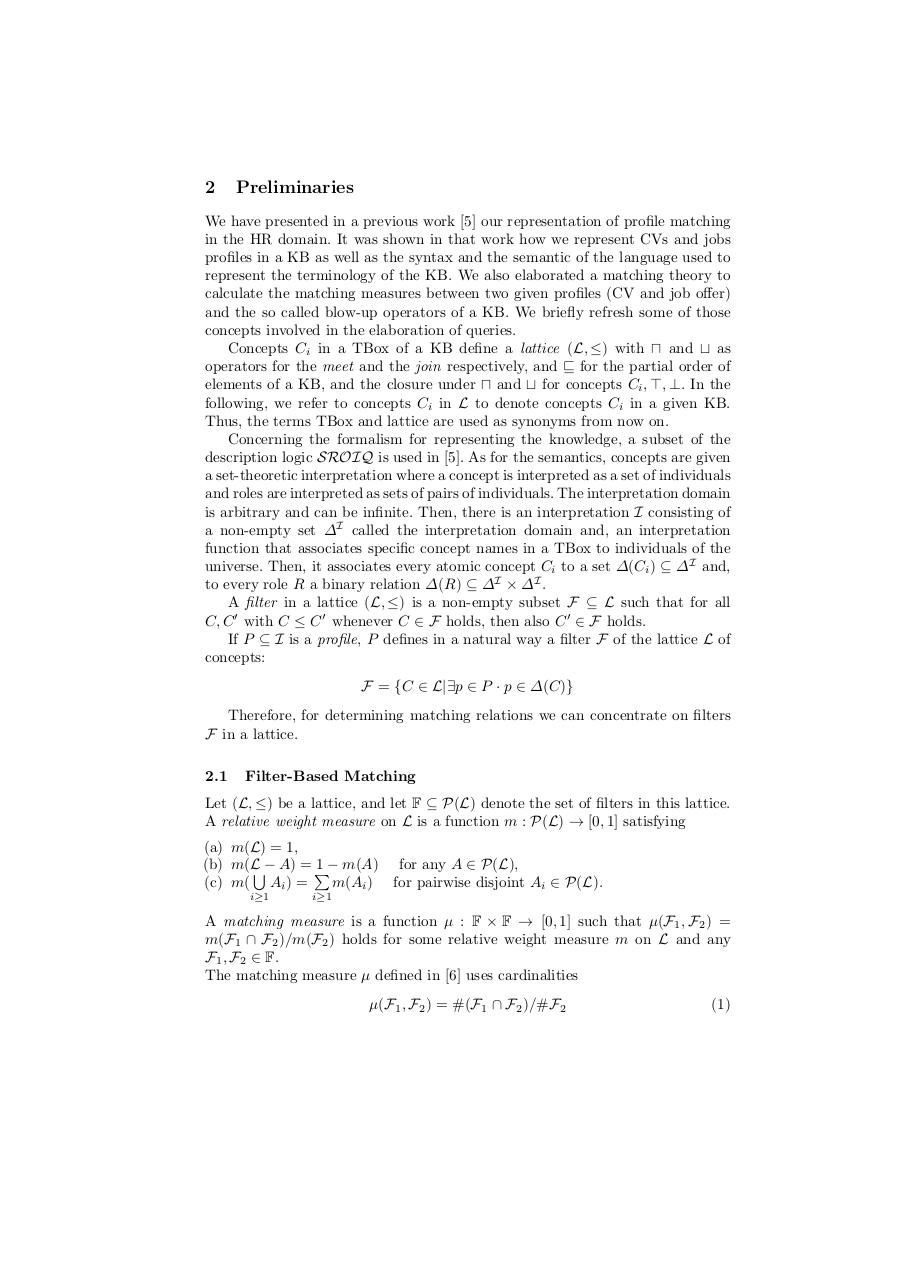# Top K Queries.pdfPage 1 2 34515

#### Text preview

2

Preliminaries

We have presented in a previous work  our representation of profile matching
in the HR domain. It was shown in that work how we represent CVs and jobs
profiles in a KB as well as the syntax and the semantic of the language used to
represent the terminology of the KB. We also elaborated a matching theory to
calculate the matching measures between two given profiles (CV and job offer)
and the so called blow-up operators of a KB. We briefly refresh some of those
concepts involved in the elaboration of queries.
Concepts Ci in a TBox of a KB define a lattice (L, ≤) with ⊓ and ⊔ as
operators for the meet and the join respectively, and ⊑ for the partial order of
elements of a KB, and the closure under ⊓ and ⊔ for concepts Ci , ⊤, ⊥. In the
following, we refer to concepts Ci in L to denote concepts Ci in a given KB.
Thus, the terms TBox and lattice are used as synonyms from now on.
Concerning the formalism for representing the knowledge, a subset of the
description logic SROIQ is used in . As for the semantics, concepts are given
a set-theoretic interpretation where a concept is interpreted as a set of individuals
and roles are interpreted as sets of pairs of individuals. The interpretation domain
is arbitrary and can be infinite. Then, there is an interpretation I consisting of
a non-empty set ∆I called the interpretation domain and, an interpretation
function that associates specific concept names in a TBox to individuals of the
universe. Then, it associates every atomic concept Ci to a set ∆(Ci ) ⊆ ∆I and,
to every role R a binary relation ∆(R) ⊆ ∆I × ∆I .
A filter in a lattice (L, ≤) is a non-empty subset F ⊆ L such that for all
C, C ′ with C ≤ C ′ whenever C ∈ F holds, then also C ′ ∈ F holds.
If P ⊆ I is a profile, P defines in a natural way a filter F of the lattice L of
concepts:
F = {C ∈ L|∃p ∈ P · p ∈ ∆(C)}
Therefore, for determining matching relations we can concentrate on filters
F in a lattice.
2.1

Filter-Based Matching

Let (L, ≤) be a lattice, and let F ⊆ P(L) denote the set of filters in this lattice.
A relative weight measure on L is a function m : P(L) → [0, 1] satisfying
(a) m(L) = 1,
(b) m(LS− A) = P
1 − m(A) for any A ∈ P(L),
m(Ai ) for pairwise disjoint Ai ∈ P(L).
(c) m( Ai ) =
i≥1

i≥1

A matching measure is a function µ : F × F → [0, 1] such that µ(F1 , F2 ) =
m(F1 ∩ F2 )/m(F2 ) holds for some relative weight measure m on L and any
F1 , F2 ∈ F.
The matching measure µ defined in  uses cardinalities
µ(F1 , F2 ) = #(F1 ∩ F2 )/#F2

(1)